Minimizing Blocking Probability in Elastic Optical Networks by Varying the Bandwidth Granularity Based on Optical Path Fragmentation | OMICS International
Journal of Lasers, Optics & Photonics

# Minimizing Blocking Probability in Elastic Optical Networks by Varying the Bandwidth Granularity Based on Optical Path Fragmentation

Al-Tarawneh L* and Taebi S

Royal Scientific Society, Amman, Jordan

*Corresponding Author:
Al-Tarawneh L
Royal Scientific Society
Amman, Jordan
Tel: +962 6 534 4701
E-mail: [email protected]

Received date: January 16, 2017; Accepted date: February 23, 2017; Published date: March 18, 2017

Citation: Al-Tarawneh L, Taebi S (2017) Minimizing Blocking Probability in Elastic Optical Networks by Varying the Bandwidth Granularity Based on Optical Path Fragmentation. J Laser Opt Photonics 4:149. doi: 10.4172/2469-410X.1000149

Copyright: © 2017 Al-Tarawneh L, et al. This is an open-access article distributed under the terms of the Creative Commons Attribution License, which permits unrestricted use, distribution, and reproduction in any medium, provided the original author and source are credited.

Visit for more related articles at Journal of Lasers, Optics & Photonics

#### Abstract

Elastic optical networks (EONs) based on orthogonal frequency-division multiplexing (OFDM) are considered a promising solution for the next optical network’s generation. It basically makes it possible to choose an adequate portion of the available spectrum to satisfy the requested capacity. In this paper, we consider the impact of spectrum fragmentation along the optical single/multipath routing transmission on the efficiency of the EONs. This involves reducing the fragmentation effects by dynamically updating and controlling the minimum bandwidth allocation granularity (g).We adopt linear and nonlinear dynamic mechanisms, which are denoted as LDAɡ and NLDAɡ, respectively, to choose proper band width granularities that are proportional to the optical link/path bandwidth fragmentation status. In order to avoid neither splitting the capacity request over many routing paths, which would increase the management complexity, nor encouraging single path transmission, the proposed schemes aim to choose a proper bandwidth allocation granularity (g) of a predefined set of suggested values. Simulation results show that varying the bandwidth granularity based on the optical path fragmentation status can offer improved performance over fixed granularity with respect to the bandwidth blocking probability, the number of path splitting, the throughput, and differential delay constraint issue in terms of: the network bandwidth utilization and multipath distribution.

#### Keywords

Optical OFDM-based networks; Networks; Routing and assignment algorithms; Fragmentation; Off-line and On-line computation problems

#### Introduction

The current optical transport network based on wavelength optical networks (OTN-WDM) suffers from serious limitations because of the expected capacity crunch in the physical capacity of the current optical fibers [1-3]. Moreover, in addition to the dramatic increase in the network services for new generations of bandwidth consuming applications (in the presence of fixed rate and rigid frequency grid of the wavelength-routed optical networks 50 GHz), the mismatch of granularity between the cooperative layers (Client-layer and WDMlayer) results in degrading the efficiency of the spectral utilization over the current OTN . Usually, most of the service requests would be provided with more than the necessary spectral resources due to the mismatch of granularity.

In order to tackle these limitations, a new generation of flexible or Elastic Optical Networks (EONs) need to be adopted .

The concept of EON was first presented in ref. . In which, the operators manage the OTNs by adaptively allocating spectral resources. In EONs, the principle of optical orthogonal frequency-division multiplexing (O-OFDM) has been considered a promising technique for the optical network future because of its helpful characteristics . Using OFDM for EON offers a finer granularity mechanism, which makes it possible to slice off the right amount of available spectrum resources to save more spectrum in order to serve other clients . In this technique, all subcarriers are overlapping in frequency domain, where each frequency subcarrier represents the smallest frequency slot (FS) that can be sliced from the available bandwidth .

The problem of routing and wavelength assignment (RWA), in a conventional optical network, has been changed to routing and spectrum allocation (RSA) which is also extended to be routing, modulation, and spectrum allocation (RMSA). Both RSA and RMSA are considered NP-hard problems [10,11]. To achieve optimum solution and reduce the complexity, this problem can be separated into two sub-problems: routing and spectrum allocation .

Various RSA mechanisms over EONs have been investigated as single path and multipath routing [10,13,14]. For instance , a novel approach as the RSA algorithm was proposed based on a distance adaptive modulation technique. It has considered a BW-efficient RMSA, whereas the lowest required number of available contiguous slots on the shortest path is allocated to accommodate the light path request. Zuqing et al.  presented a HSMR-OPC (hybrid single-/ multi-path routing using online path computation). Fixed bandwidth allocation granularities (?) are used in their algorithms. Their results showed that choosing an appropriate value of the BW allocation granularity (?) would be an important parameter that the operator can control to reduce the number of rejected service requests.

Although, the EON is considered as a promising solution for conventional rigid optical networks limitations, a serious fragmentation problem arises. After some time, the spectrum on the optical links/ paths will be fragmented because of the non-uniform spectrum usage , which definitely would degrade the spectrum utilization, spectrum efficiency that would achieve elasticity benefits and increase the blocking probability as well. Minimizing fragmentation and defragmentation’s methods are considered a serious challenge in RSA problems as well as RMSA.

In this paper, we aim to reduce the fragmentation’s problem effect by dynamically controlling and updating the bandwidth granularity for each candidate optical path in single and multipath provisioning routing mechanism. A tradeoff between management complexities (multipath routing) and reducing the blocking probability (single path routing) have been taken into account. The details of this work are described in the next sections.

#### Dynamic Minimum Bandwidth Allocation

Granularity and fragmentation

Dynamic minimum bandwidth allocation granularity: The minimum spectrum that can be assigned over an optical path represents the minimum bandwidth allocation granularity (?). That is to say, the optical path is considered an acceptable candidate path if it only has at least this granularity size of the predefined bandwidth. The ? is defined as a block of slots which is an optical spectrum of contiguous subcarriers. In EONs, these subcarrier frequency slots (FSs) should meet the spectrum contiguity, spectrum continuity, and nonoverlapping constraints along the requested optical paths.

In our presented model, we dealt ? as a variable size of minimum bandwidth that could be assigned based on the spectrum availability and the fragmentation status over the candidate optical path. A hybrid single/multipath routing (HSMR) is activated to choose a proper optical path(s) by either assigning a single path or splitting the request among multipath routing. To avoid service request blocking, the ? will be updated dynamically to make sure that each fragmented spectrum could be utilized efficiently. However, at the same time, the service request capacity would not be splitting over many paths: C=Σi Ci where C denotes the total requested capacity and is the sub-capacity that can be assigned for an optical candidate path. Assigning a small bandwidth granularity, for example ?=1, at the transponders to meet the minimum required unit of slots, would lead to spread out the requested capacity over many paths. This definitely will increase the management complexity at the receiver side. On the contrary, choosing large ? would lead to a single path transmission scenario which would increase the blocking probability.

In terms of fragmentation, a high fragmentation ratio (γ) over an optical path would reduce the probability of finding an adequate number of continuous subcarrier slots that obey the routing constraints. Meanwhile, the low fragmentation ratio would increase the probability of finding more of the consecutive subcarriers.

Fragmentation: In an operational network, while the time passes, some of the light path requests will depart as soon as their life time is finished; new requests will arrive and will need to be accommodated. The available spectrum would be highly fragmented in the case of highly dynamic service provisioning. Each one of the new arrivals will need a certain amount of spectrum in the form of contiguous and continuous frequency slots (FSs); otherwise, it will be rejected (blocked).

The connection arrivals and disconnections are a purely random process, which means the spectrum distribution over the optical paths is random as well. This randomness of the fragmentation makes it hard to determine the optimum value of the best BW allocation granularity. However, due to the fragmentation over optical links/paths, the nonaligned or isolated contiguous subcarriers in the spectrum domain of EON, are considered useless resources. To increase the spectrum utilization in the network, the network’s operator would utilize the fragmentation status over the network too.

Bandwidth fragmentation assessment has been widely investigated in literature, generally, in terms of bandwidth blocking probability (BBP) [14,16-19]. Prior research aims at reducing the fragmentation’s effect by either proposing spectral defragmentation algorithms or managing the demand’s connections over the network. For instance , the results illustrated the importance of choosing a proper BW allocation granularity (?) in impacting both the blocking BBP and the number of path splitting per request. In ref.  simulation and mathematical modeling showed that there is a strong relationship between the bandwidth fragmentation and the bandwidth allocation granularity (?). Other researches aim at calculating the bandwidth fragmentation ratio (γ), which has been formulated in various research papers such as in ref. . In Al-Tarawneh and Taebi , a link, a path and network utilization entropy (UE_ path) of assessing resource fragmentation was proposed, as indicated in Equation 4.

To mitigate the fragmentation’s effect along the optical link

(e) And the candidate optical path (Pi), which belongs to the main sets E and P, respectively; we need to optimize the spectrum utilization and the spectrum efficiency by taking into account the following objective:

Minimize ΣeεE,?εp FSi

Minimizing the equation above, based on routing constraints, requires managing the bandwidth granularity (?) to allocate proper spectrum along the optical routing path. We believe that serving the network with certain bandwidth granularities need to be managed carefully in terms of optical path fragmentation status.

In this work, we are focusing on the role of granularity (?) that can be played in general as a control parameter to increase the spectral efficiency, thereby reducing in an overall the blocking opportunity, the number of path splitting and other networks’ parameters.

#### Proposed Mechanism

We propose two algorithms in order to change the minimum BW granularity (?) adaptively. Both of them depend on the currently available spectrum and the fragmentation status over the optical candidate’s path (Pi). The first one is the linear dynamic adaptation mechanism denoted as LDA?; meanwhile, the other is a non-linear dynamic adaptation granularity denoted as NDAg. To evaluate the performances, we adopted the following two equations,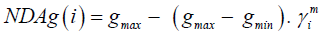(2)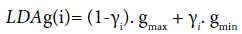(3)

In Equation 2, the m represents the mth order of the curve, m ≥ 2, see Figure 1. Increasing m results in maintaining the slow adaptation for low fragmentation ratio (γ). Because the fragmentation ratio is usually small at this early stage, it is not necessary to assign a small granularity, nor activate the multipath provisioning, which reduces the management complexity. This factor would be left to the operator to choose based on the network specifications. We used m=2 for evaluation purposes. In order to reduce the unlimited choices of NDAg(i) and LDA?(i), we constrained the range of ? into five integer values which are {1,2,3,4,5}, as indicated in refs. [14,20,21]. In Equations 2 and 3, stands for the fragmentation ratio over the optical path Pi. The fragmentation ratio is calculated by the path utilization entropy  by the following equation: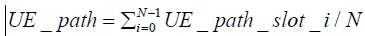(4)

N is the number of total frequency slots in link e, and ∈ [0,1]. The i represents the candidate path among the P possible paths in multipath provisioning for a light path request LR(s,d,C) where Pi ∈ P and P={P1,P2,..Pi}.

We used the HSMR-OPC/-FPS (Hybrid Single/Multipath Routing– Online Path Computation- First Path Set) schemes that are proposed  to investigate our suggested adaptation mechanisms. The requested capacity (C) of light path request (LR) splits over multiple paths if no one path could accommodate the requested capacity. The distributed sub-capacities should satisfy the following equation: (Figure 1)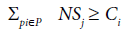(5)

Each requires contiguous slots for each Pi candidate path. In terms of NS, NG (guard band), and the slot’s bandwidth as a function of modulation level format, the can be rewritten as follows: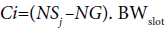(6)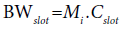(7)

Where represents the digital modulation level format of the optical transmission reach which is given as follows:

Mi =Length (Pi)    (8)

The maximum number of the candidate’s path of the set P is limited by |k|, max (number of candidates Pi) ≤ |k|, however, k is the k-shortest fiber optical path between the source (s) and the destination (d). To reduce the complexity of the computations and to make this approach feasible in practice, we limited |k| to be 5 shortest candidate paths. This complexity includes checking the k-shortest path, the subcarrier slots (FS), and the fiber links (E), so the computational complexity of applying the algorithm is in O(|k|.|FS|.|E|).

The network topologies have been represented by graph (G) of G(V,E,B,D), where, E, B, and D are the node set, the fiber link set, the bandwidth, and the length of e ( where e belongs to E set), respectively. While the network is operational and updated after each operation, the G topology is converted to a new virtual auxiliary topology G\ (V,E,D\). In G\ any link that doesn’t have the minimum bandwidth granularity (?) will be omitted. Each link in G\ will be re-weighted as follows: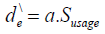(9)

Where α is a modulation constant and Susage is spectrum usage with respect to the total spectrum, these parameters were defined as mentioned in :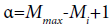(10)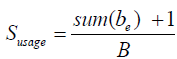(11)

Where Mmax represents the maximum modulation level format. Sum (be) is the spectrum usage over the link e, B is the total bandwidth. In our research the minimum available ?=1 over any link should be 1 to stay in the updated auxiliary graph.

Routing and spectrum allocation constraints

Contiguousness of spectrum constraint: The allocated subcarrier slots (FSs), for the whole demand in a single path transmission or the part of the demand in multipath transmission, needs to be beside each other on the candidate optical path (s). To make sure of that, Sp, e, i is defined as a binary variable, it equals 1 if the of the link e of the path (Pi) is used and otherwise equals 0., is defined as an integer variable representing the number of an available free spectrum (gaps), to make sure that the link has at least one free available slot, we determine the total number of gaps along the e, so that we use the following equation: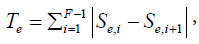(12)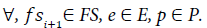If Te ≥ 1, then based on the counted gaps and their locations over the link (e), a mask is created that can be defined as follows: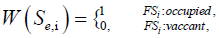(13)

Then, we create another mask, for a candidate path, basically, is used to determine the spectrum pattern over the candidate path depending on the that includes the gaps location along the optical path. After that we just need to find the proper consecutive spectrums location We and Wp,i are used to define the spectrum locations in addition to preventing the spectrum from overlapping.

Continuity of spectrum constraint: There is no frequency conversion or regeneration over the network. Each allocated frequency for any service demand over the link (e) should be allocated over the same locations over all the links that belong to the assigned optical path. Each allocated frequency will not be released until the expiration of the service time. To guarantee the spectrum continuity, we used the following equations: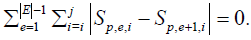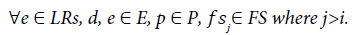(14)

Spectrum non-overlapping constraint: Ensures that there are assigned subcarrier slots over a physical link (e), where ∈ and e work as part of the assigned optical path (p), which can’t be assigned for another spectrum optical path even though the latter would share the same physical link e. So here we applied the same idea that is mentioned  to avoid overlapping as follows: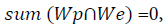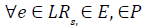(15)

Where ∩ represents the AND operator and sum is to add all bits.

Differential delay constraint: The differential delay issue, during multipath provisioning, is due to the fiber optics’ nature, the transmitted signals would experience a differential delay effect . We investigated the under the presence and the absence of the differential delay issue.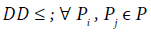(16)

is the differential delay: is a threshold which represents the propagation delay time delay between Pj(t) and Pi(t), respectively. Where can be calculated as follows: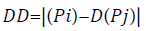(17)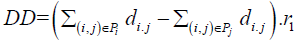(18)

Equation 18 represents propagation delay in terms of the path's lengths. r1 stands for a propagation delay in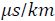which is considered 5as mentioned in different works such as [23,24]. Delay that is caused by equipment is out of the scope of this work.

Network topologies and simulation parameters of the test networks: To test the performance of our approaches for dynamic bandwidth granularity adaptations, NSFNET and US backbone network topologies are used, as shown in Figures 2A and 2B. These topologies have been employed as a test bed by other works, such as in refs. [14,20,21]. NSFNET has 14 stations indexed from 1 to 14 with 22 links, and the US backbone network has 24 stations, from 1 to 24 with 43 links.

These network topologies have been represented by the (V, E, B, D). The light path request is represented by (s, d, C, Δt), where s, d, C, and Δt are the source, the destination, the capacity, and the service time, respectively. The arrivals of rate λ requests per time unit are generated by Poisson processes. The service time Δt of each request followed the negative exponential distribution with mean μ time units , and the traffic load in Erlangs can be considered as ( λ* μ).

We propose heuristic algorithms for on-line path computations in algorithms 1 and 2, respectively. The algorithms show the procedures of proposed dynamic adaptation schemes. Algorithm 1 proposes the dynamic adaptation mechanism where we can use either LDA? or NDA? granularity that is given by Equations 2 and 3. Table 1 shows the simulations parameters which have been used in simulations (Figures 2 and 3).

Simulation Parameters
Frequency slots number per link, B 300 slots
Frequency slot Bandwidth, BWslot Capacity of a frequency slot, Cslot 12.5 GHz
12.5 GHz
Requested Capacity (C) Range: 12.5 -200 Gb/s
Slots number for guard-band,NGB 1
?, bandwidth allocation granularity 1-5
Transmission Reach of :
9600 km,
4800 km,
2400 km, and 1200 km.
Modulation format :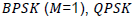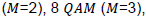and,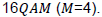Path candidates number, K 5
Propagation delay (r)
DD: Differential Delay.
5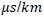10 ms

Table 1: Shows the simulation parameters which have been used in simulation.

Algorithm 2 is considered an extension of Algorthim1 where the differential delay constraint is activated. Algorithm 3 is used for fixed path calculations. We use these algorithms to investigate the adaptation’s effect on the overall network performance mainly in terms of BBP and other parameters such as network fragmentation, throughput, distribution of path splitting per request, and bandwidth network utilization.Mechanism using online path computation

Input: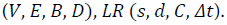Output: Single or HSMR for LR

receive the incoming service request LR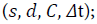While the network is operational. Update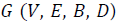to be a virtual auxiliary topology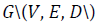using (Equation 9);

1. sort the calculated k-shortest routing path from s to d in G\

based on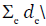4. for all candidate paths do

5. Choose the modulation level for real distance, using (Equation 8);

6. Calculate UE_path (γi) for each path using (Equation 4);

7. Determine the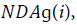using (Eq 2);

8. for all consecutive slots with size ≥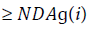do

9. use (Equation 6) to allocate capacity as in (Equation 5);

10. if is satisfied in (5)

11. break for-loops in step 4 and step 8;

12. end if

13. end for

14. end for

15. if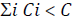then

16. assign the request as blocked request;

17. end if

18. end whileInput: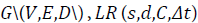and a differential delay threshold (τ )

Output: All candidate paths that satisfy. Condition: no available single short path

1. while the network is operational do

2. for =1 to K, do

3. sort all available paths in ascending order of delay in new path set P

5. end for

6. for =1 , do

7. If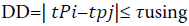(Equation 18) then

8. as paths can be assigned

9. end if

10. end for

11. use (Equation 6) to allocate capacity as in (Equation 5);

12. If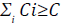13. assign the request as blocked request

14. end if

15. end whileInput: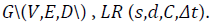Output: Single or HSMR for LR

1. for all that ask for service do

2. find K-shortest routing path and Implement a set of path

3. end

While the network is operational. Update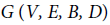5. sort the calculated k-shortest routing path from s to d based on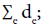6. for all candidate paths do

7. Choose the modulation level for real distance, using (Equation 8);

8. Calculate UE_path (γi) for each path using (Equation 4);

9. Determine the NDA?(i ), using (Equation 2);

10. For all consecutive slots with size ≥ DA?(i) do

11. use (Equation 5) to allocate capacity Ci as in (Equation 6);

12. if C is satisfied in (Equation 5)

13. break for-loops in step 4 and step 8;

14. end if

15. end for

16. end for

17. If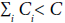then assign the request as blocked request;

18. end if

18. end if

Validation and numerical simulation results

To evaluate the dynamic granularity adaptation performance of both LDAg(i) and NDAg(i) under some of the network’s parameters, we used online path computation of a hybrid single/multipath routing based on dynamic RMSA (HSMR-OPC). We also used fixed path sets with dynamic RMSA using HSMR-FPS-LSoSHF (LSoSHF: Largest slots-over-square-of-hops first) to investigate the number of path splitting per request. We simulated the spectrum allocation policy for ?max=5 and ?min=1, which are static values. Our adaptation mechanisms are embedded, and the multipath provisioning part is activated when there is no single path that can accommodate the light path request LR(s,d,C). Figure 2 shows that the bandwidth blocking probability is improved significantly by using dynamic BW granularity adaptation for both test beds compared to using a fixed ?max=5 and, therefore, approaches that of ?min=1.

Figure 4 illustrates the simulation results with respect to the average throughput. HSMR-RMSA-OPC of ?=1, 5, LDAg(i) and NDAg are presented. The average throughput of the LDAg and NDAg are enhanced compared to the fixed minimum granularity; ?=1. It is evident that LDAg and NDAg show improvement for all traffic loads in terms of traffic throughput. Average throughput is improved greatly for ? compared to ?=5. This improvement of about 5 Tb/s is beneficial to the performance of optical networks. Average throughput is improved greatly for NDAg compared to ?max=5. This improvement of about 5 Tb/s is beneficial to the performance of optical networks. (Figures 2-4)

In terms of path splitting, as in Figure 5, NDAg offers an improvement for the distribution percentage when compared to ?=1. Simulation data show that 80% of the total requests are still served by a single routing path by using the NDAg compared to 75% using ?min=1 and 93% for ?max=5. The largest number of path splitting per request for ?max=5, NDAg and ?min=1 are 5, 6 and 12, consecutively. The simulation results showed that using a larger BW allocation granularity of ? (i.e., ?=5) forces the HSMR scheme to become a single path routing scenario, which explains the increase in BBP.

Figure 6 presents the simulation results on average network bandwidth utilization as a function of traffic load in Erlangs for HSMR-OPC- NDAg with/without differential delay constraint (DD), fixed bandwidth granularity (MP-FFSA with ?=5) with DD, and the single path first fit spectrum allocation (SP-FFSA). It can be seen that NDAg without the DD scheme achieves the best network bandwidth utilization compared to the others. However, in spite of about a 10% reduction (on average) in utilization (at high traffic load) occurring when adding the DD constraint ? is also able to maintain a higher utilization than both MP-FFSA and SP-FFSA.

Also, it is clear that at traffic loads less than 300 Erlangs there is no noticeable differentiation among the schemes, possibly because there are a lot of gaps which reflect the spectrum availability along the links and the paths. At 1000 Erlangs, NDAg achieves 12.5 % and 30% better than MP-FFSA and SP-FFSA, respectively.

Figure 7 shows a multipath distribution based on path-difference using NDAg with DD as a function of a traffic load for different differential delay constraints. The path distance differences were considered as 0 km, 1000 km, 2000 km, and 3000 km which are 0 ms, 5 ms, 10 ms, respectively. For example, at a traffic load of 1000 Erlangs, 87% of the multipath routing is served by 0 differential delays. In ref.  well-controlled management is achieved within 3000 km or within 15 ms; meanwhile, the NDAg achieves better in a well-controlled management within 2000 km or within 10 ms (Figure 6 and Table 2).

Traffic Load (Erlangs) g=1 NDAg g=5
400 0 0 0
500 0 4.76 23.7
600 0 5 24.05
700 0 12.7 23.33
800 10.3 19 23.37
900 13.75 20.69 23.53
1000 13.75 20.92 29.47
1100 14.67 21.47 29.72
1200 15.52 21.21 31.42
Average (%) 14 15

Table 2: Percentage increase in Bbp for with/without Dd constraints.

The BBP for NDAg and other schemes with or without DD constraints are shown in Figure 8. It can be noticed that considering the DD has increased the BBP.

When the load traffic increases, the BBP also rises too. Table 2 illustrates the percentage increase for with or without DD constraints for different traffic loads. With or without the fixed ?=1 and ?=5, the BBP increases by about 14% and 25% on average. Meanwhile, the NDAg with or without DD constraint has increased by around 15%.

Comparisons for a traffic load of 1000 Erlangs using the simulation results show that NDAg with DD is about 21.5% higher than NDAg without DD, as well as 14% and 29.5% for ?=1 and ?=5, respectively. For ?=1, there is no significant differentiation for traffic loads less than 800 Erlangs. The last column shows the calculated average for each bandwidth granularity mechanism ?=1, NDAg, and ?=5.

#### Conclusion

In sum, a dynamic BW allocation granularity adaptation mechanisms, denoted as LDAg and NDAg, were proposed and defined. The performance of these schemes was tested and validated using MATLAB simulation software. They were investigated under dynamic path algorithms to show the effect of granularity adaptation on the EON’s performance metrics specifically the BBP performance. The simulation results show that LDAg and NDAg schemes allow improved performance over using a fixed minimum BW allocation granularity scheme that uses fixed granularities such as ?=1 and ?=5. A fixed-path selection (FPS) algorithm was also investigated to show the NDAg effect on the path splitting per request. The dynamic adaptation reduces the number of path splitting per request compared to the scheme that uses the smallest fixed granularity (?=1).

Differential delay effects on the multipath service provisioning were also discussed. The simulation results showed that the adaptation of NDAg offers improved performance in terms of “distribution of the path-distance differences” compared to single path service.

For future analysis, it would be a good idea to improve these mechanisms by taking into account other network metrics. A promising step towards realizing fully-optimized BW allocation is considering defragmentation optimization and differential delay during multipath provisioning.

#### References

Select your language of interest to view the total content in your interested language

### Article Usage

• Total views: 1788
• [From(publication date):
March-2017 - Dec 14, 2019]
• Breakdown by view type
• HTML page views : 1656Can't read the image? click here to refresh